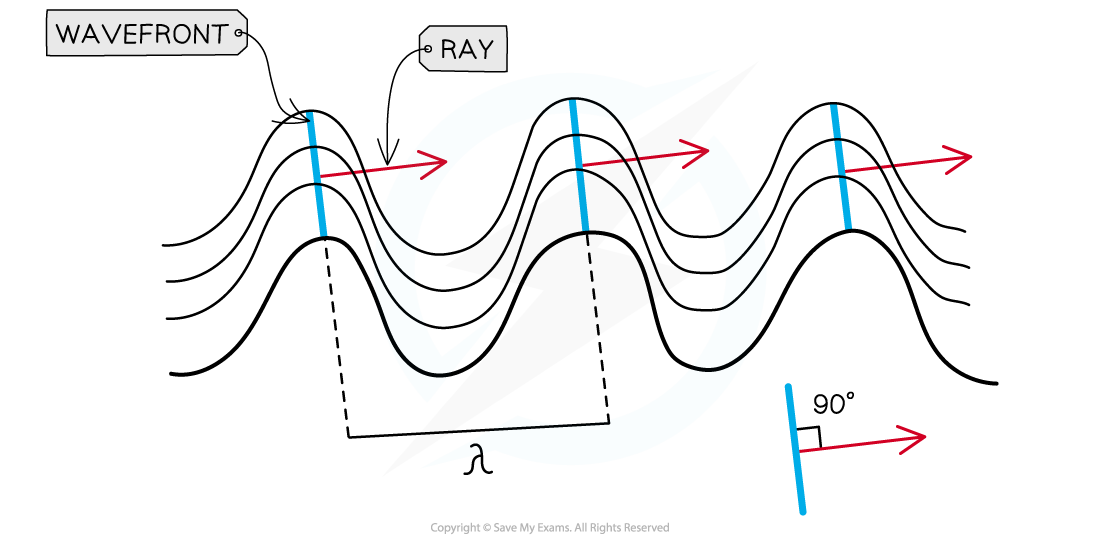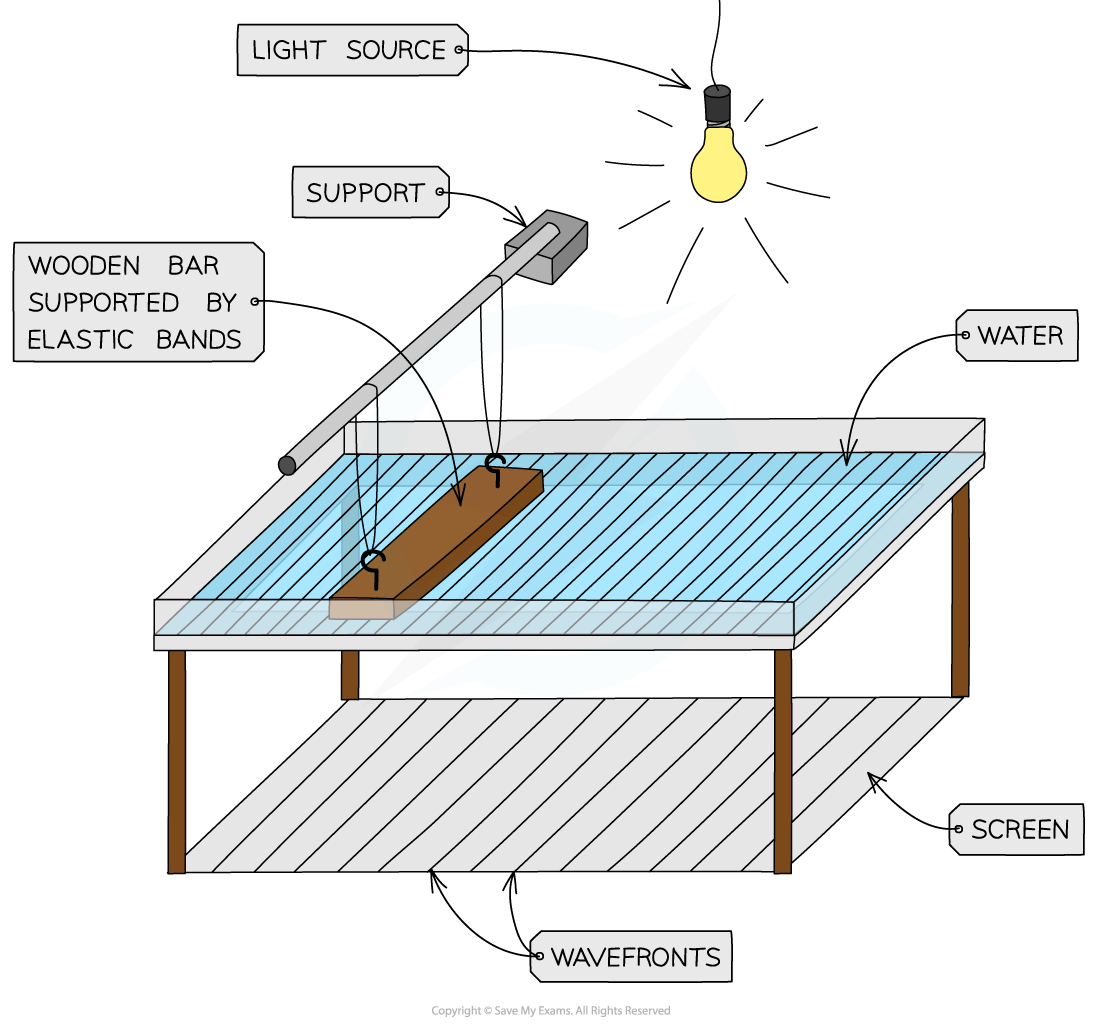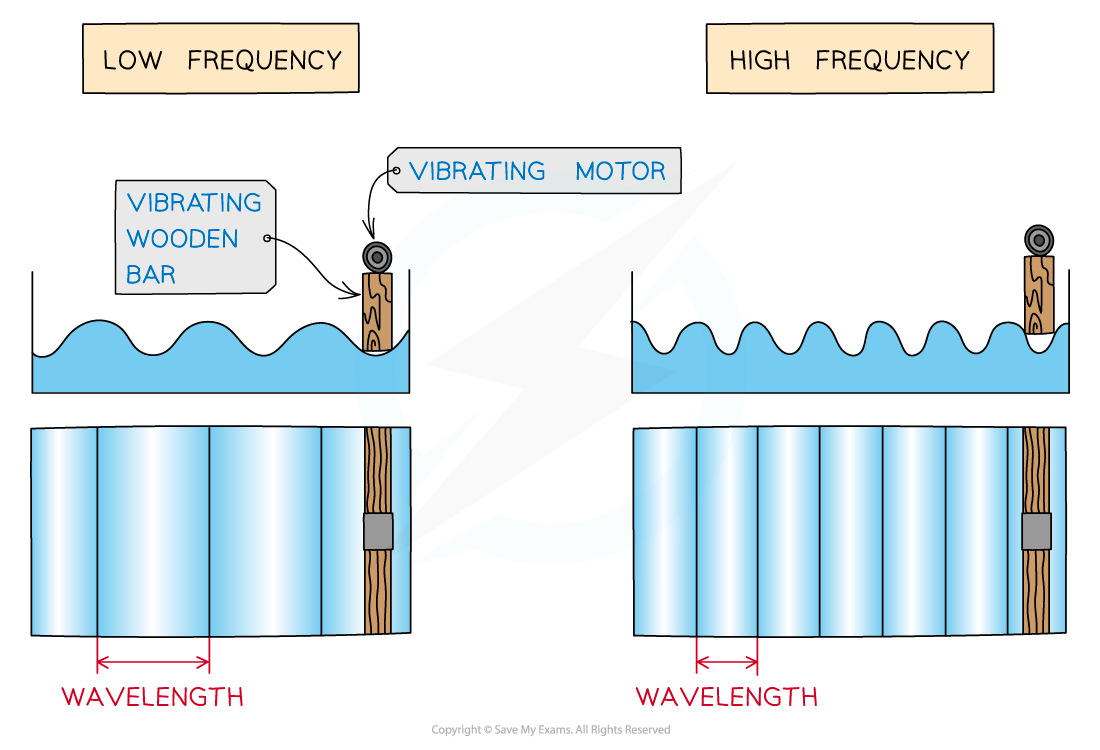# IB DP Physics: SL复习笔记4.3.1 Wavefronts

### Wavefronts

• Waves can be represented graphically in two different ways:
• Wavefronts - lines joining all the points that oscillate in phase and are perpendicular to the direction of motion (and energy transfer)
• Rays - lines showing the direction of motion (and energy transfer) of the wave that are perpendicular to the wavefrontWavefronts and rays for transverse waves travelling in a horizontal plane

• The distance between successive wavefronts is equal to the wavelength of the waves
• Ripple tanks are used a common experiment to demonstrate diffraction of water wavesWave effects may all be demonstrated using a ripple tank

• The diagram below shows how the wavelengths differ with frequency in a ripple tank
• The higher the frequency, the shorter the wavelength
• The lower the frequency, the longer the wavelengthRipple tank patterns for low and high frequency vibration